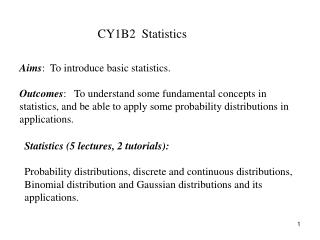# CY1B2 Statistics - PowerPoint PPT PresentationDownload PresentationCY1B2 Statistics

CY1B2 StatisticsDownload Presentation## CY1B2 Statistics

- - - - - - - - - - - - - - - - - - - - - - - - - - - E N D - - - - - - - - - - - - - - - - - - - - - - - - - - -
##### Presentation Transcript

1. CY1B2 Statistics Aims: To introduce basic statistics. Outcomes: To understand some fundamental concepts in statistics, and be able to apply some probability distributions in applications. Statistics (5 lectures, 2 tutorials): Probability distributions, discrete and continuous distributions, Binomial distribution and Gaussian distributions and its applications.

2. Statistics • The probability theory and statistics is a mathematical representation of random phenomena. • Random variable • The outcome of a random phenomenon may take a numerical value. When the outcomes of an event that produces random results are numerical, the numbers obtained are called random variables. • Random variable has probabilities associated with the various values of the variable. • Discrete random variable has a countable number of possible values.

3. Probability • probability is always taken as a number lying between 0 and 1 and is denoted by p(x) • p(x) = 1 means that an event is certain to happen • p(x) = 0 means that an event is certain NOT to happen • so a toss of a coin could be represented as • P(heads) = 0.5 and P(tails) = 0.5 • or, more formally • p(x) = 0.5 x = heads, tails

4. Rules of probability • there are some rules associated with probabilities when the events are not dependent on each other • P(A or B) = P(A + B) = P(A) + P(B) • P(A and B) = P(AB) = P(A)P(B) • for example, rolling a dice multiple times, or rolling two dice • if the events are not dependent but they are not mutually exclusive then • P(A + B) = P(A) + P(B) - P(AB) • how can this be extended to more than two possible events?

5. Discrete probability distributions (i) Binomial distribution • An experiment which follows a binomial distribution will satisf the following requirements (think of repeatedly flipping a coin as you read these): • The experiment consists of n identical trials, where n is fixed in advance. • Each trial has two possible outcomes, S or F, which we denote ``success'' and ``failure'' and code as 1 and 0, respectively. • The trials are independent, so the outcome of one trial has no effect on the outcome of another. • The probability of success, p, is constant from one trial to another.

6. (i) Binomial distribution The probability in a process with two outcomes; p for one outcome (e. g. success), q for another (e. g. failure) (q=1-p) The probability of r successes in n trials is given by the (r+1)th term of the binomial expansion of Where n! = 1 ×2×3×… ×n, factorial of n.

7. is the count of choosing r from n. The coefficients of polynomial expansion can be listed as a pyramid as n=0; 1 n=1: 1 1 n=2; 1 2 1 n=3; 1 3 3 1 n=4; 1 4 6 4 1 n=5; 1 5 10 10 5 1 ………………………

8. Example: Toss a coin 4 times, p =q=0.5, n=4. Find the probabilities of throwing 0,1,2,3,4 heads. A plot of p(r) versus r is called a probability distribution. The Figure on the left is the binomial probability distribution for this example (p=q=0.5) (Symmetrical)

9. Example: Toss a dice 4 times, Find the probabilities of throwing 0,1,2,3,4 sixs. (p=1/6, q=5/6) The Figure on the left is the binomial probability distribution for this example (p=1/6, q= 5/6) (not symmetrical)

10. The mean value (average number of successes) The mean square value can be shown to be The variance is a measure of the deviation from the mean, or the width of the distribution can be shown to be

11. The square root of the variance ,σ, in known as the standard deviation. Example: Random sample of 900 people are asked “ have you heard of Cybernetics’’. Find mean, mean square and variance of the expected distribution, assuming that over the whole population, 1/3 would say “yes’’. 2/3 would say “no”.